## A short review of the visibility complex

We consider polygonal scenes and maximal free segments.

To represent them, we'll use a duality transform. A line y=ax+b is associated with the point (a,-b).

The set of lines going through a point is a line in the dual space.

The visibility complex is a partition of the maximal free segments according to the edge of a polygon they touch.

A face is a connected component of segments which see the same objects.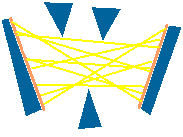scene dual space

It is bounded by edges which are segments tangent to one object.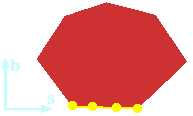scene dual space

The vertices correspond to the bitangents of the scene.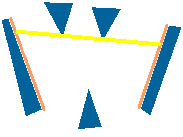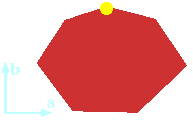scene dual space

Many segments can be colinear. Thus we have to add a third dimension which is not continuous.

For example, the segments on the right and on the left of the triangle share the same (a, b) coordinates but have different z values.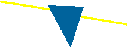scene dual space

The visibility complex is a 2D cellular complex immersed in 3D.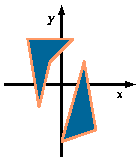scene dual space

Here we can see the vertices.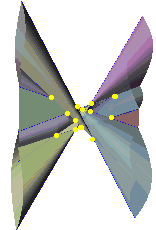scene dual space

And a dual line corresponding to a point in the scene.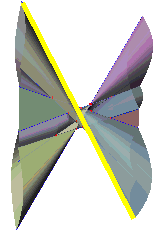scene dual space

Next : Form Factors